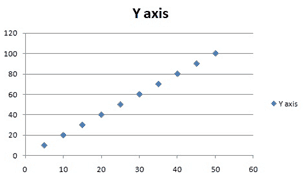# MCQ – 79342

### Question

A graph is given below. Calculate the regression equation.A.

X = 2Y + X

B.

X = Y + 2*X

C.

X = Y/2

D.

Y = X/2